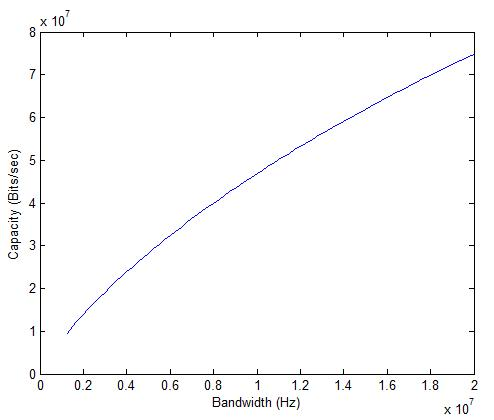# Shannon Capacity of LTE (Ideal)

Shannon Capacity of LTE in AWGN can be calculated by using the Shannon Capacity formula:

C=B*log2(1+SNR)

or

C=B*log2(1+P/(B*No))

The signal power P is set at -90dBm, the Noise Power Spectral Density No is set at 4.04e-21 W/Hz (-174dBm/Hz) and the bandwidth is varied from 1.25MHz to 20MHz.It is seen that the capacity increases from about 10Mbps to above 70Mbps as the bandwidth is varied from 1.25MHz to 20MHz (keeping the signal power constant). It must be noted that this is the capacity with a single transmit and single receive antenna (MIMO capacity would obviously be higher).#### Author: John (YA)

John has over 20 years of Research and Development experience in the field of Wireless Communications. He has worked for a number of companies around the world including Qualcomm Inc. USA.

0.00 avg. rating (0% score) - 0 votes

## 7 thoughts on “Shannon Capacity of LTE (Ideal)”

1.phil says:

So, channel capacity is relatively higher with narrow bandwidths.

Is this why LTE has lots of narrow resource blocks and sub-carriers?

1.John says:

Capacity does not change by dividing the frequency band into narrower frequency bins (keeping the SNR to be the same for each bin). Narrower frequency bins make the task of an equilizer easy since the frequency response over a frequency bin can be assumed to be constant.

2.John says:

Yes Satya you are right. I have made the correction. Thank you!

3.Satyabrata Mohanty says:

If I am not wrong, above plot is inbetween Capacity-Bandwidth rather than Capacity-SNR.

Kindly see to it.

4.Satyabrata Mohanty says:

Sir, Thanks

SNR = P/(B*No)

Keeping P and No constant, SNR or SNR(dB) will decrease with increase in B.

Of course, with increase in B, capacity increases giving capacity-bandwith plot correctly as plotted above.
But, capacity-SNR(dB) plot is coming otherwise. Since, in this case, capacity increases even if SNR(dB) decreases because of increased B.

5.John says:
6.ymf says:

Hi John,

Thanks for the post. Just a quick question, if Shannon’s Theorem was modelled in a Time-Invariant Channel using a channel realization matrix H with SNR = (P*|H|^2)/(noise variance), how will I obtain a capacity curve if I was to have fixed signal power P?
Currently I am varying P in order to obtain a curve similar to yours, with H being a random 1×1 (or 2×2) complex valued matrix.